calmer than you are

# brainfuck optimization strategies

Mats Linander
2015-01-07 - New York

In almost every computation a great variety of arrangements for the succession of the processes is possible, and various considerations must influence the selection amongst them for the purposes of a Calculating Engine. One essential object is to choose that arrangement which shall tend to reduce to a minimum the time necessary for completing the calculation.”

Lovelace’s words still ring true, almost two centuries later. One way to choose that arrangement is to use an optimizing compiler. In this post, we’ll have a look some important compiler optimization strategies for the brainfuck programming language and we’ll examine how they affect the run time of a handful of non-trivial brainfuck programs. The chart below illustrates the impact of the techniques covered.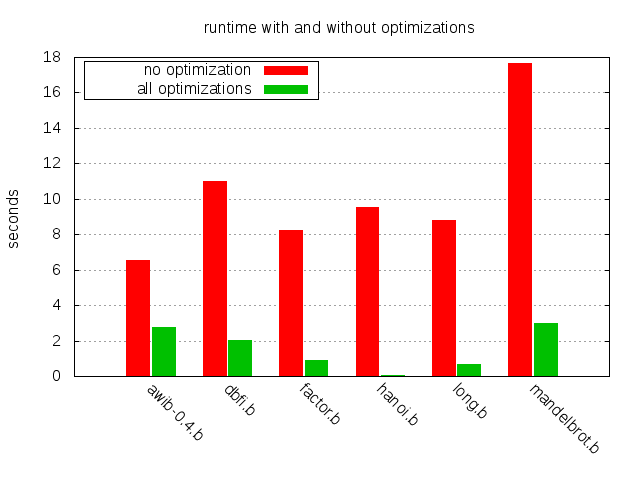# Prerequisites

If you’re new to the brainfuck programming language, then head on over to the previous post where we cover both the basics of the language as well as some of the issues encountered when writing a brainfuck-to-java compiler in brainfuck. With that said, most of this post should be grokkable even without prior experience of the language.

### Programs worth optimizing

In order to meaningfully evaluate the impact different optimization techniques can have, we need a set of brainfuck programs that are sufficiently non-trivial for optimization to make sense. Unsurprisingly, most brainfuck programs out there are pretty darn trivial, but there are some exceptions. In this post we’ll be looking at six such programs.

The interested reader is encouraged to head over to this repository for a more detailed description of these programs.

### An intermediary representation

Our first step is to define an intermediary representation (IR) on which an optimizer can operate. Working with an IR enables optimizations that are platform independent and brings more expressiveness than brainfuck itself. To further illustrate how the IR functions, we’ll also give C implementations for each operation.

In the table below we find all 8 brainfuck instructions, their IR counterparts and an implementation of the IR in C. This basic mapping provides a very simple and somewhat naive way of compiling brainfuck.

BF IR C
+ Add mem[p]++;
- Sub mem[p]--;
> Right p++;
< Left p--;
. Out putchar(mem[p]);
, In mem[p] = getchar();
[ Open while(mem[p]) {
] Close }

The C code depends on the memory area and pointer having been set up properly, e.g. like this:

char mem = {0};
int p = 0;


At this point the IR is no more expressive than brainfuck itself but we will extend it later on.

## Making things faster

Throughout the remainder of this post we’ll look at how different optimizations affect the execution time of our 6 sample programs. The brainfuck code is compiled to C code which in turn is compiled with gcc 4.8.2. We use optimization level 0 (-O0) to make sure we benefit as little as possible from gcc’s optimization engine. Run time is then measured as average real time over 10 runs on a Lenovo x240 running Ubuntu Trusty.

### Contraction

Brainfuck code is often riddled with long sequences of +, -, < and >. In our naive mapping, every single one of these instructions will result in a line of C code. Consider for instance the following (somewhat contrived) brainfuck snippet:

+++++[->>>++<<<]>>>.


This program will output an ascii newline character (ascii 10, ‘\n’). The first sequence of + stores the number 5 in the current cell. After that we have a loop which in each iteration subtracts 1 from the current cell and adds the number 2 to another cell 3 steps to the right. The loop will run 5 times, so the cell at offset 3 will be set to 10 (2 times 5). Finally, this cell is printed as output.

Using our naive mapping to C produces the following code:

mem[p]++;
mem[p]++;
mem[p]++;
mem[p]++;
mem[p]++;
while (mem[p]) {
mem[p]--;
p++;
p++;
p++;
mem[p]++;
mem[p]++;
p--;
p--;
p--;
}
p++;
p++;
p++;
putchar(mem[p]);


We can clearly do better. Let’s extend these four IR operations so that they accept a single argument x indicating that the operation should be applied x times:

IR C
Add(x) mem[p] += x;
Sub(x) mem[p] -= x;
Right(x) p += x;
Left(x) p -= x;
Out putchar(mem[p]);
In mem[p] = getchar();
Open while(mem[p]) {
Close }

Applying this to our snippet produces a much more compact piece of C:

mem[p] += 5;
while (mem[p]) {
mem[p] -= 1;
p += 3;
mem[p] += 2;
p -= 3;
}
p += 3;
putchar(mem[p]);


Analyzing the code of our six sample programs is encouraging. For instance, about 75% of the instructions in factor.b, hanoi.b and mandelbrot.b are contractable, i.e. part of a sequence of at least 2 immediately adjacent >, +, < or -. Lingering around 40% we have dbfi.b and long.b, while awib-0.4.b is at 60%. Still, we shouldn’t stare ourselves blind at these figures; it could be that these sequences are only executed rarely. Let’s look at an actual measurement of the speedup.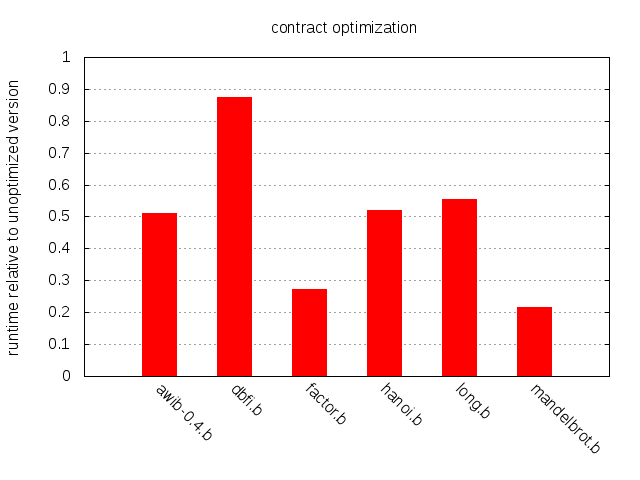The impact is impressive overall and especially so for mandelbrot.b and factor.b. On the other end of the spectrum we have dbfi.b. All in all, contraction appears to be a straightforward and effective optimization that all brainfuck compilers should consider implementing.

### Clear loops

A common idiom in brainfuck is the clear loop: [-]. This loop subtracts 1 from the current cell and keeps iterating until the cell reaches zero. Executed naively, the clear loop’s run time is potentially proportional to the maximum value that a cell can hold (commonly 255).

We can do better. Let’s introduce a Clear operation to the IR.

IR C
Add mem[p]++;
Sub mem[p]--;
Right p++;
Left p--;
Out putchar(mem[p]);
In mem[p] = getchar();
Open while(mem[p]) {
Close }
Clear mem[p] = 0;

In addition to compiling all occurrences of [-] to Clear, we can also do the same for [+]. This works since (all sane implementations of) brainfuck’s memory model provides cells that wrap around to 0 when the max value is exceeded.

Inspecting our sample programs reveals that roughly 8% of the instructions in hanoi.b and long.b are part of a clear loop, while the corresponding figure for the other programs is around 2%.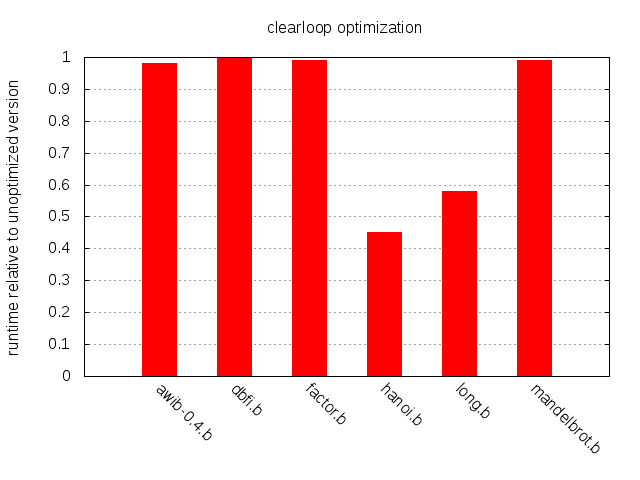As expected, the impact of the clear loop optimization is modest for all but long.b and hanoi.b, but the speedup for these two is impressive. Conclusion: the clear loop optimization is simple to implement and will in some cases have significant impact. Thumbs up.

### Copy loops

Another common construct is the copy loop. Consider for instance this little snippet: [->+>+<<]. Just like the clear loop, the body subtracts 1 from the current cell and iterates until it reaches 0. But there’s a side effect. For each iteration the body will add 1 to the two cells above the current one, effectively clearing the current cell while adding its original value to the other two cells.

Let’s introduce another IR operation called Copy(x) that adds a copy of the current cell to the cell at offset x.

IR C
Add(x) mem[p] += x;
Sub(x) mem[p] -= x;
Right(x) p += x;
Left(x) p -= x;
Out putchar(mem[p]);
In mem[p] = getchar();
Open while(mem[p]) {
Close }
Clear mem[p] = 0;
Copy(x) mem[p+x] += mem[p];

Applying this to our copy loop example ([->+>+<<]) results in three IR operations, Copy(1), Copy(2), Clear, which in turn compiles into the following C code:

mem[p+1] += mem[p];
mem[p+2] += mem[p];
mem[p] = 0;


This will execute in constant time while the naive implementation of the loop would iterate as many times as the value held in mem[p].Improvement across the board, except for dbfi.b.

### Multiplication loops

Continuing in the same vein, copy loops can be generalized into multiplication loops. A piece of brainfuck like [->+++>+++++++<<] behaves a bit like an ordinary copy loop, but introduces a multiplicative factor to the copies of the current cell. It could be compiled into this:

mem[p+1] += mem[p] * 3;
mem[p+2] += mem[p] * 7;
mem[p] = 0


Let’s replace the Copy operation with a more general Mul operation and have a look at what it does to our sample programs.

IR C
Add(x) mem[p] += x;
Sub(x) mem[p] -= x;
Right(x) p += x;
Left(x) p -= x;
Out putchar(mem[p]);
In mem[p] = getchar();
Open while(mem[p]) {
Close }
Clear mem[p] = 0;
Mul(x, y) mem[p+x] += mem[p] * y;While most programs benefit slightly from the shift from Copy to Mul, long.b improves significantly and hanoi.b not at all. The explanation is simple: long.b contains no copy loops but one deeply nested multiplication loop; hanoi.b has no multiplication loops but many copy loops.

### Scan loops

We have so far been able to improve the performance of most of our sample programs, but dbfi.b remains elusive. Examining the source code reveals something interesting: out of dbfi.b’s 429 instructions, a whopping 8% can be found in loops like [<] and [>]. Contrast that with awib-0.4 being at 0.8% and the other programs having no occurrences of this construct at all. These pieces of code operate by repeatedly moving the pointer (left or right, respectively) until a zero cell is reached. We saw a nice example in the previous post of how they can be utilized.

It should be mentioned that while scanning past runs of non-zero cells is a common and important use case for these loops, it is not the only one. For instance, the same construct can appear in snippets like +<[>-]>[>]<, the exact meaning of which is left as an exercise for the reader to figure out. With that said, the scanning case can be very time consuming when traversing large memory areas and is arguably what we should optimize for.

Fortunately for us, the problem of efficiently searching a memory area for occurrences of a particular byte is mostly solved by the C standard library’s memchr() function. Scanning “backwards” is in turn handled by the GNU extension memrchr(). In a nutshell, these functions operate by loading full memory words (typically 32 or 64 bits) into a CPU register and checking the individual 8-bit components in parallel. This proves to be much more efficient than loading and inspecting bytes one at a time.

Let’s extend the IR by adding two new operations: ScanLeft and ScanRight.

IR C
Add(x) mem[p] += x;
Sub(x) mem[p] -= x;
Right(x) p += x;
Left(x) p -= x;
Out putchar(mem[p]);
In mem[p] = getchar();
Open while(mem[p]) {
Close }
Clear mem[p] = 0;
Mul(x, y) mem[p+x] += mem[p] * y;
ScanLeft p -= (long)((void *)(mem + p) - memrchr(mem, 0, p + 1));
ScanRight p += (long)(memchr(mem + p, 0, sizeof(mem)) - (void *)(mem + p));

An unfortunate consequence of using array indexes instead of direct pointer manipulation is that the C versions of the scan operations look pretty horrid. Well, such is life.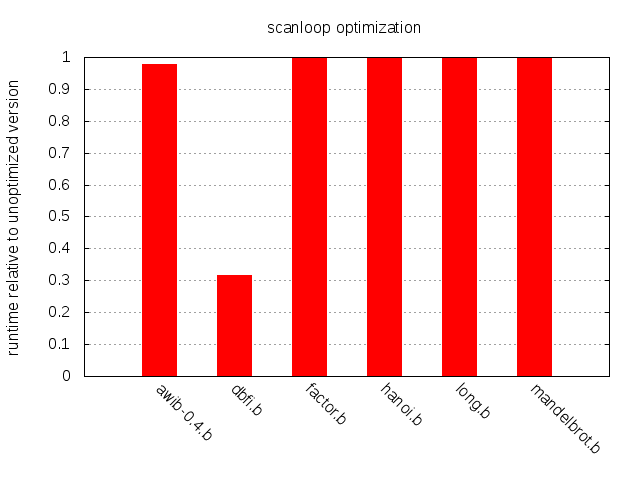More than a 3x speedup for dbfi.b while the other programs see little to no improvement. There is of course no guarantee that memchr() will perform as well on other libc implementations or on a different architecture, but there are efficient and portable memchr() and memrchr() implementations out there so these functions can easily be bundled by a compiler.

### Operation offsets

Both the copy loop and multiplication loop optimizations share an interesting trait: they perform an arithmetic operation at an offset from the current cell. In brainfuck we often find long sequences of non-loop operations and these sequences typically contain a fair number of < and >. Why waste time moving the pointer around? What if we precalculate offsets for the non-loop instructions and only update the pointer at the end of these sequences?

IR C
Add(x, off) mem[p+off] += x;
Sub(x, off) mem[p+off] -= x;
Right(x) p += x;
Left(x) p -= x;
Out(off) putchar(mem[p+off]);
In(off) mem[p+off] = getchar();
Clear(off) mem[p+off] = 0;
Mul(x, y, off) mem[p+x+off] += mem[p+off] * y;
ScanLeft p -= (long)((void *)(mem + p) - memrchr(mem, 0, p + 1));
ScanRight p += (long)(memchr(mem + p, 0, sizeof(mem)) - (void *)(mem + p));

Note that while Clear and Mul are included in this table, the test was run with the operation offset optimization in isolation, so these two operations were never emitted by the compiler.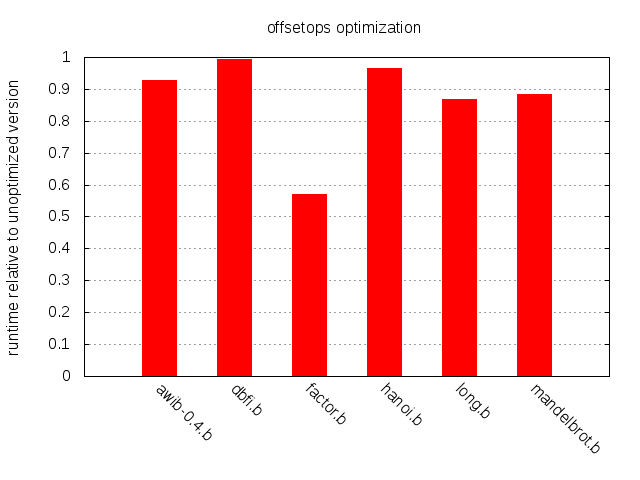Tangible results, albeit somewhat meager.

### Applying all optimizations

To wrap up, let’s have a look at what we can accomplish by applying all these optimizations to our sample programs.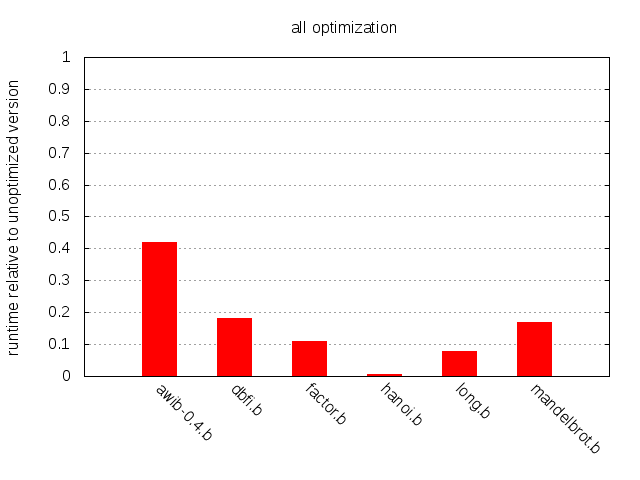Significant improvement across the board, ranging from about 130x in the case of hanoi.b to the 2.4x speedup of awib-0.4.

## Summary

Fairly simple techniques proved to significantly improve the performance of our sample programs. Brainfuck compiler writers should consider implementing at least some of them. Now, spending time and effort on such an esoteric language as brainfuck may seem like a pointless exercise, but we hope and believe that some of the concepts can carry over to more real settings. Also, mucking around with brainfuck is just plain fun.

That was all.